# Required grip pressure

Dave Tutelman  --  July 2, 2020

How tightly does a golfer have to grip the club in order to make a swing? I was asked this question by a reader, and the answer was more interesting than either of us expected.

In early July of 2020, I got the following email from Chris Daigle.

In a subsequent note, he wrote:
Chris, you're closer than you give yourself credit for. The calculation of centripetal force is central to the whole thing. But there are other factors as well. Let's see what they are.

## Overview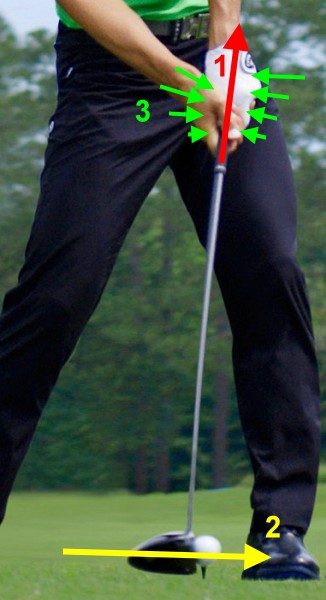The problem statement is, "How much grip pressure do I need for my swing?" Golf instruction tells us that that we don't want more tension in the hands and forearms than is absolutely necessary. But how much is necessary? There's a traditional analogy, usually attributed to Sam Snead, to hold the club "like you were holding a baby bird in your hands". Poetic for sure, but is it realistic? Let's find out how much grip pressure you need just to do the job of swinging your club at V miles per hour. That has to be the bare minimum grip pressure that works. And we don't want to use too much more than the bare minimum, because tension in the hands and forearms interferes with release.

The first question we must answer is: at what point in the swing does the maximum force requirement occur? The intuitive and obvious answer is: just before impact, where the maximum angular velocity of the club requires hands to exert the maximum centripetal force to hang onto the club. We will proceed in this vein, and I believe it gives the most useful answer. But at the end of this article, we will discover a point in the swing that may call for an even larger hand force -- though not one that involves keeping the club in the hands.

Given that assumption, there are three things we have to concern ourselves with:
1. The centripetal pull we have to exert just to keep the club on its rotational path, and not let it fly out of our hands. This depends on clubhead speed and some of the club's dimensions. This peaks out at or near impact, which is why the pictures is shown just before impact.
2. Any additional force we need to continue to accelerate the clubhead near impact.
3. The hand forces on the handle of the club to resist the forces from #1 and #2.
In the body of the article, we will deal with them in this order. Then we sanity-check our results against some real-world data:
1. What sort of grip force can the hands actually exert?
2. What grip forces have actually been measured during the downswing.
The results are interesting, to say the least.

### Executive summary

We will first find F, the centripetal force the hands need to exert on the club to keep it from flying down the fairway. Then we will discover that we can neglect the possibility of an additional force to accelerate the clubhead in the vicinity of impact. Finally, we look at the mechanisms by which the hands can exert an axial force on the club shaft; they consist of friction and taper. Applying these mechanisms to the formula for F, we get the following expression for G, the total force in pounds the hands exert on the grip.

 G  = 0.00129 V2  μs R (M + m/2) [1 - sin(a)]

Where:
• V is the clubhead speed, in miles per hour.
• μs is the static coefficient of friction between the hands and the handle.
• R is the distance from the mid-hands point to the center of mass of the clubhead, in inches.
• M is the mass of the clubhead, in grams.
• m is the mass of the shaft, in grams.
• a is the angle of taper of the grip where the hands are applying the force.

Other topics covered include relating dynamometer readings to the ability to apply grip pressure, and comparing our results to some measured grip forces during the downswing.

## Centripetal force

### Separate out the angular component

The clubhead travels on a curved path. It requires a force perpendicular to the path to keep it from flying off on a straight line -- thus flying completely out of the golfer's grip. This perpendicular force is called a centripetal force.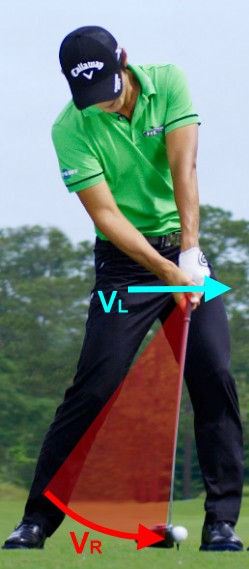Centripetal force depends on the curvature of the path. Its usual formulation is:
 F  = m v2 R
Here the curvature is represented by the radius of curvature R.

But we have to remember that the clubhead's velocity is due to two effects: the angular velocity of the club (hence the emphasis on centripetal force) and the linear velocity of the hands' motion. On the diagram (built on top of a photo from the Howard's Golf web site), we show these two velocities, which add together to create the clubhead speed. But only VR, the velocity due to rotation, contributes to the centripetal force the hands must exert. How can we separate out VL, so only VR contributes to the centripetal force?

For good quality swings, somewhere between 12% and 20% of the clubhead speed depends on the linear hand speed VL. Actually, that variation is mostly from study to study, not from golfer to golfer; the results of any one study seem to come out within a range of a couple of percentage points across the golfers. So let's arbitrarily use 15%, an 85-15 split between speed due to rotation of the club and speed of the hands. That means 85% of clubhead speed can be attributed to rotation of the club, and contributes to the centripetal force. We will calculate the centripetal force by using VR and the obvious radius in the diagram. That system can be superimposed on the linearly translating hands, but only the rotational component gives the force exerted on the hands system -- which is what we are looking for.

A word about the radius R. We are using the radius in the translating frame, so we are only dealing with pure angular motion. That radius is from the center of the hands (the "mid-hands point") to the clubhead CG, so it is a few (3 to 4) inches less than the length of the club itself.

Now we can find the force due to the clubhead speed V, the clubhead mass M, and the radius R.

 F  = M (.85 V)2 R = 0.723 M V2 R

### Centripetal force due to the shaft

That covers the force due to the clubhead. But the shaft has mass and is rotating. So there must be a centripetal force required to keep the shaft from flying off on its own -- just like the clubhead. The clubhead was easy, because we treated it as a point mass at a distance from the mid-hands. But the shaft's mass is distributed more or less uniformly from the mid-hands to the clubhead, and must therefore be treated as a distributed mass.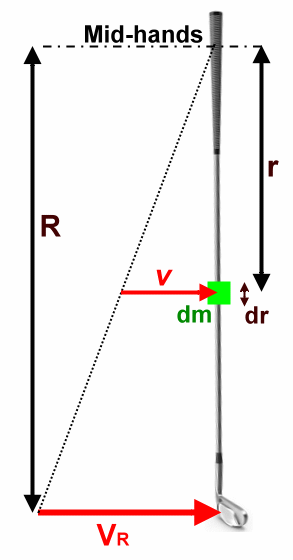You won't miss anything but the math if you skip this note.

That turns this into a calculus problem -- a simple calculus problem, but one we need to formulate in calculus terms.

As per the diagram, we are going to integrate (add together) the centripetal force due to all the infinitesimal lengths of shaft mass dm between the mid-hands point and the tip.

 F  = R ∫ r=0 dm v2 r

We will make the assumption that the mass of the shaft (which we call m) is uniformly distributed along its length. This is seldom the case, but is close enough so the answer will not have a large error. Therefore, we write:

 dm  = m R dr

Which gives us:

 F  = m R R ∫ r=0 v2 r dr

The velocity v at distance r from the mid-hands is proportional to r.

 v  = r R VR

Which gives us:

 F  = m VR2 R3 R ∫ r=0 r  dr

Evaluating the integral and applying the range gives

 F  = m VR2 R3 [ ½ r2 R ] r=0

 F  = m VR2 2 R

This is a reasonable and intuitively satisfying result. It says that the force is half what it would be if the shaft's mass were a point mass at R. In fact, it is possible to generalize that a bit. If the mass of the shaft is not uniformly distributed, the fraction is not one half, but rather the distance from the hands to the balance point divided by R. (Just so I don't forget how I derived it, I recorded the derivation in the Appendix.)

### Centripetal force: the bottom line

Thus the centripetal force to keep the entire club from flying out of the hands is:

 F  = 0.723 V2 R (M + m/2)

That is great as far as it goes. But there remains the small issue of measurement units. The equation demands a consistent set of units, such as meters/kilograms/seconds (MKS) or feet/slugs/seconds. But those are not the units that come to mind if you're an American clubmaker or even American golf instructor. We are more comfortable and intuitive in terms of:
• F in pounds. (Good so far.)
• V in miles per hour. (Not feet per second.)
• R in inches. (Not feet.)
• M and m in grams. (Not slugs nor even pounds.)
That is just a complete scramble of units. Let's see if we can find the conversion factors to make the units come out right. If we do it well, it should just show up as a coefficient different from the current 0.723.

Let's start by explicitly including a conversion multiplier to each of our quantities. Since we want the force in pounds, let's use the foot/slug/second system, since its unit of force is the pound.
 Multiply by ↓ to convert ↓ into ↓ 1.47 miles per hour feet per second 1/12 = 0.0833 inches feet 1/14594 grams slugs

 F  = 0.723 * 1.472 V2 .0833 R (M + m/2) 14594

Multiplying it out, we get our formula for centripetal force.

 F  = 0.00129 V2 R (M + m/2)

This looks like the sort of formula we were hoping for. Let's compute it for a few different clubs:

 Club Driver graphite shaft Driver steel shaft 5-iron steel shaft Club length 45.5 44.5 38 R 42 41 34.5 Head mass M 200 200 256 Shaft mass m 50 115 120 Head speed V ↓ Centripetal force (pounds) ↓ 10 1 1 1 20 3 3 5 30 6 7 11 40 11 13 19 50 17 20 30 60 25 29 43 70 34 40 58 80 44 52 76 90 56 66 96 100 69 81 118 110 84 98 143 120 100 117 170 130 117 137 200 140 135 159 232 150 155 182 266

This is certainly the same order of magnitude that the conventional wisdom would lead us to expect. There is a graph of this table in the Appendix.

 The next question we have to deal with is, how much additional force does the golfer exert to keep the clubhead accelerating through impact?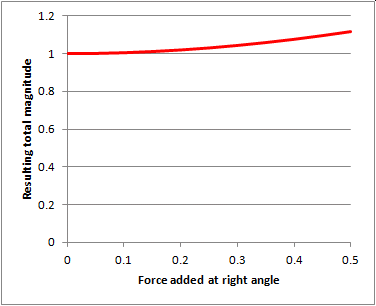For golfers who have acceleration going beyond impact, how are they accelerating the clubhead? In particular how are they accelerating the angular velocity? I single that out because linear acceleration along the hand path requires forces along the hand path -- at a right angle to the major centripetal force we already have. Unless they are major forces indeed, they do not add noticeable magnitude to the grip force required. The graph at the right shows how little effect a small force has on the magnitude of the total force when added at a right angle to a larger force. Both the added force and the resulting total are normalized to a unit force. So even if we have a right angle force of half the centripetal force, it only increases the total force by 12%. So unless we are looking for rather precise results, we can ignore forces along the hand path when looking for the total "pull" on the hands. Let's move on to forces that can cause angular acceleration. Many good golfers do continue their angular acceleration right up to and including impact. How do we know? Because biomechanics studies show a positive net torque on the club, applied by the hands, even at impact. Let's look at what two such studies tell us.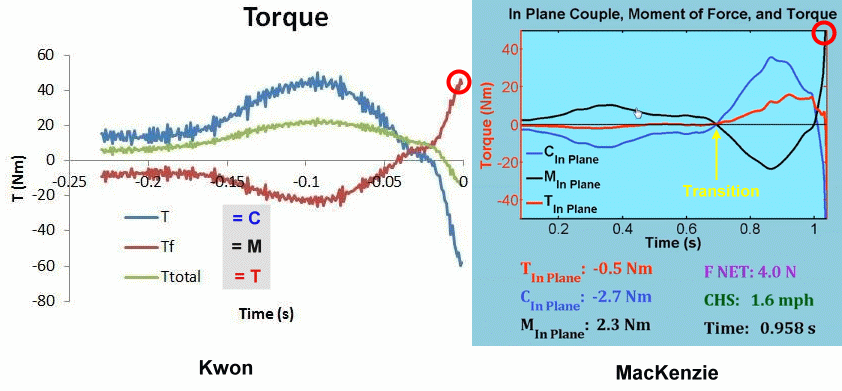These are graphs of the in-plane ("alpha") torque during the swing up until impact. They look different, but are really quite similar. The one on the left was posted by Dr Young-Hoo Kwon as part of a tutorial in the Golf Biomechanists group on Facebook in 2015; it may still be available there. It shows three curves: The hand couple. The moment of the hand force. The total torque, the sum of 'a' and 'b'. The one on the right is from a video screenshot of a video by Dr Sasho MacKenzie. It shows the same three curves, but with different names. The gray shaded area on the Kwon graph is a translation of Kwon's notation to Sasho's. The curves look different, but that difference can be explained by the fact that Sasho's graph includes the backswing. If you just look at the downswing, the curves are really very similar.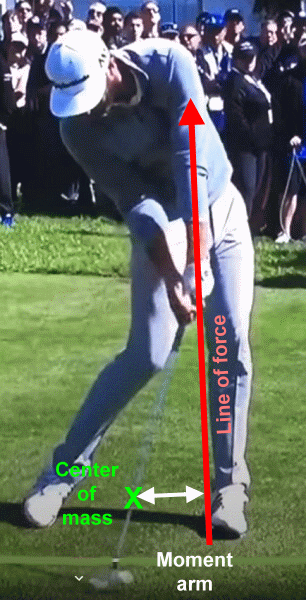The most significant similarity (important to us, for this discussion) is the moment of the hand force at impact. It is about 50 Newton-meters on both graphs. Let us see what the moment of the hand force means. This image shows Dustin Johnson at the moment of impact. His lead (left) arm is pulling up along its length. We don't know this for a fact, but it is very likely because: The centripetal force at the speed he generates is substantial -- over 100 pounds. The mechanism to generate a force along the length of the arm is much more powerful than is needed to generate a forward or backward force along the hand path. As biomechanics studies show us, there is no need to use forces other than along the arm, at least not in the vicinity of impact. So let's assume the line of force he applies to the grip is along the left arm itself. But that is not the line of the shaft! The club is lagging considerably behind the line of force. In fact, that is where the angular acceleration comes from. The "moment of force" that creates all that clubhead speed is due directly to the torque represented by that [red] force time the moment arm, the distance between the line of force and the center of mass of the club. (Engineers call the center of mass the "center of gravity", and it is almost the same place as what clubmakers call the "balance point" of the club.) The basics of physics say that a force on a body not through the center of mass (CoM) generates a torque on the body. The torque is equal to the magnitude of the force times the distance the line of force is from the CoM. We can see that this torque will create angular acceleration to increase the angular velocity of the club -- which is exactly what we should want to do. It is this moment of force, and not the hand couple, that accelerates the club during the last 50-100msec of the swing. I chose a picture of Dustin Johnson (as opposed to some other golfer) because it gave me the most easily understood diagram; few other golfers, if any, have the line of force leading the CoM by as much as he does. This can look very different from golfer to golfer. I tried an interesting calculation: What force would it take to generate 50 Newton-meters of torque given any particular golfer's moment arm at impact. I estimated the moment arm for a half-dozen pictures, and got anywhere from 55 to 220 pounds of force. This rather neatly brackets the sort of numbers we got for centripetal force. For professional golfers, we can expect the driver clubhead speed to be 110-125mph, giving a centripetal force of 85-110 pounds. What should that tell us? My conclusion is not tightly reasoned, but seems (intuitively, to me, at least) to be a good working hypothesis. Top-notch golfers apply all the centripetal force they can, and get their clubhead speed from keeping the line of that force as far ahead of the club as they can. The way a Dustin Johnson generates big clubhead speed is to keep his pulling force further ahead of the club than other golfers can.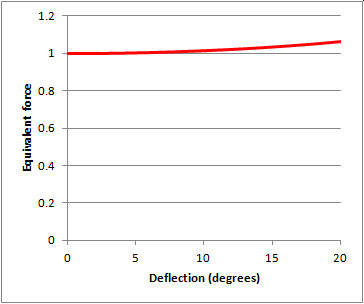So I will provisionally state that the force exerted by these guys is nearly the centripetal force, but deflected backwards to move the extended line of the force forward of the club's CoM. When you deflect a force, you turn it into two component forces: a slightly smaller force in the original direction, plus a component perpendicular to the original direction. We can do a similar graph to the one above, to see how much of a difference this makes in the force. The graph at the right shows how much we have to multiply our original force by to get back to the same centripetal component we had before we deflected the force. Even at 20° of deflection (what Dustin Johnson exhibits in the picture) we only have to make up 6% of the force. That is small enough to be almost negligible. It is worth noting that the camera that took the picture is not aimed perfectly perpendicular to the swing plane. Therefore, any numbers will be approximations at best. I used a plastic protractor on the computer screen to determine that the angle was 20°. The graph says there is no real requirement for precision -- which is good, because there was no real precision either. The bottom line for all this is that the centripetal force, all by itself, is a pretty good approximation to the total force that needs to be resisted by the golfer's grip pressure. It may be off by 5-10 percent (probably less), but it will give you a very good idea how much force is there to be resisted.

## Grip pressure

At this point, we know the force trying to pull the club out of our hands. The final step is to find out how much grip pressure is needed to resist this force, how much is needed to keep the club in our hands.

### Friction

Now we know almost everything we need in order to find the grip force. We know that the force has to be enough to keepthe club from pulling out of the hands; the hands have to be able to apply the full centripetal force, and maybe a few percent more. What is left is friction.

Here is a view of the club's handle being held by a golfer wearing a glove. The red arrows are the only force the golfer is able to apply directly -- squeeze the handle of the club. This action must be enough to keep the club from being pulled out of the hands by centrifugal force, which is the reaction force to the centripetal force the golfer uses to keep the club rotating in a curved path.

But the red arrows, the force the golfer can apply, are all normal (that is, perpendicular) to the direction of the centripetal force the golfer wants to apply. There is no component that can help in this case. How can a normal force create a tangential force? The answer is friction.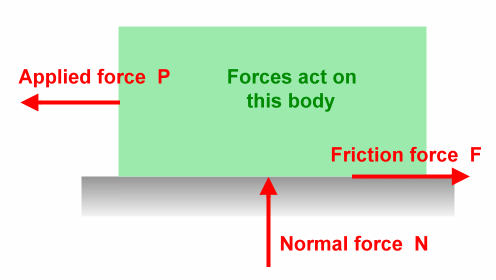Here's a diagram that, with only minor variation, is in every physics and engineering text as "Figure 1" for the chapter on friction. And it will be Figure 1 for us as well. What it shows is:
• The green body rests on the gray surface, exerting its weight as a force on the surface. The surface presses back (equal and opposite reaction) with normal force N.
• Someone wants to pull the green body horizontally along the surface, so they apply force P. If there were no friction between the body and the surface, then the body would accelerate according to P=ma.
• But there is friction. It shows up as a counter-force F, preventing the body from moving until P exceeds the maximum F that friction can impose.
That maximum F depends on the "grippiness" of the two surfaces, which is called the coefficient of static friction, μs. It gives a very simple relationship:

F  =  μs N

This seems to be the problem we are trying to solve. We have the hands exerting forces all around the handle of the club (as in the upper picture). We want those forces to prevent the club from flying out of the hands under the pull of a centripetal force that could exceed 100 pounds. So the problem would appear to be to minimize N (the grip force) for a given F (the centripetal force). In other words, we want a high friction μs between the handle and the hand or glove.

With this formulation, the goal we started with is N, the sum of all the normal forces that the hands apply to the handle of the golf club. Since we already know F, we can find out how much N is required from:

 Grip pressure (really total force on the grip)  =  N  = F μs

### Coefficient of friction for the grip

So what is the coefficient of friction for a golf grip? I found it hard to find a really good answer. Here are two of the best resources I found.
• A really good introductory article on coefficient of friction. This includes a table with μs for a large variety of materials. This table introduces the notion -- which should have been obvious with a little thought -- that a single material does not have a coefficient of friction; it is a property of a pair of materials meeting at a common surface. One of the lessons from this article is that μs for "slippery" substances runs about 0.1 more or less, and for "sticky" substances 1.0 or occasionally even more.
• A forum discussion in GOLFWRX. The question is the one we have. I did not see a definitive, authoritative answer, but most seem to be using 0.7 or so. (μs for rubber on rubber is over 1.0, so 0.7 is certainly feasible.)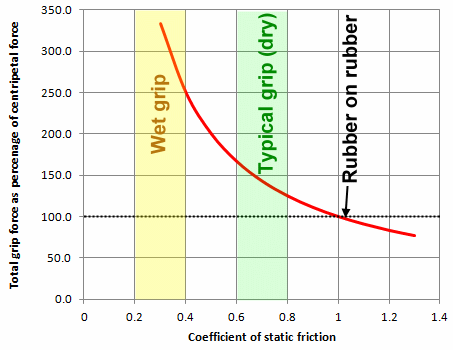There are obviously other considerations that will modify the 0.7 up or down:
• Whether or not a glove is worn. I didn't see anything in a technical discussion, but it seems from my personal experience that μs is higher if a glove is worn than playing with bare hands.
• Wet or dry. Adding moisture in the form of sweat or rain definitely reduces the coefficient of friction.
• Condition. A brand-new grip tends to be soft and tacky, an old grip hard and smooth. (There are some grip materials on the market today that I have been unable to wear out or age out noticeably; they are made by Star Grip and Pure Grips.)
To illustrate the importance of all this, let me cite a very fresh anecdote -- from today. I just got home from the golf course, where I played a round in my least favorite weather -- hot and humid. I sweat a lot, enough so a round like this can be dangerous to my health. But it is also a threat to the integrity of my golf game, because a wet interface between hands and handle has a much lower μs than a dry one. And I find it difficult to wear a glove in such weather, because the inside of the glove rapidly becomes an uncomfortable swamp.

So I was playing without a glove, and playing increasingly badly. My most common swing thought was, "Hang onto the club because it is going to slip in your hands." After completely duffing three shots on the fifteenth hole, I reached into the bag and pulled out a lonely, seldom-used glove, and pulled it onto my left hand. Then I hit the best 3-wood shot I had hit all day. I kept taking the glove off and putting it back on, so I could have a reasonable grip but not let the inside of the glove become gooey. And I left it off for short game and putting, where the required forces are low. That solved the problem. After my triple-bogey on #15, I finished bogey, par, par.

Hey, I'm in the middle of writing about this. I should have known better. But it took me until a total meltdown on the fifteenth to remember the importance of the glove in boosting the coefficient of static friction.

### Surface texture

It is fun reading patents and crazy ads in golf magazines, and one kind of off-the-wall innovation in these places is new designs and materials for golf grips and golf gloves. The point of many of these is to raise the coefficient of static friction so high that you just don't have to worry about grip pressure. For the most extreme of these inventions, the baby bird would be quite safe, but the rules of golf would be seriously jeopardized.

Here are a few of the more obvious ones, but there are plenty of them.
• A deep "tread" in the surface of the handle. This draws upon two effects. (1) A rain tire gets its gripping power from the ability to channel water into the grooves, allowing more intimate contact between the raised part of the tire and the road. If the problem is not just surface dampness but lots of water (like rain), this might also work for a golf grip. (2) If you look at what causes friction on a microscopic level, it is usually little irregularities in the surfaces grabbing one another and even locking together until the force becomes too great to resist. A deep tread goes this one better; it provide big irregularities in the surface.
• A rubber imitation of the old leather wrap grip; every grip company makes one of these. Most of them also have holes in the surface to imitate punched leather. The rationale is the same as the reason above: provide depressions to channel off rain and sweat, and provide some macro-irregularity of the surface.
• Velcro! I have seen (not recently) a matched grip and glove that gripped together with Velcro. The USGA and R&A disallowed it, of course.
• Nearly microscopic plastic ball and socket pairs on matching grip and glove. Like Velcro, this has been disallowed.
• A glove that could bring the coefficient of static friction up to 1.5. It dates to 2016, and I have no idea whether it has been ruled conforming, nor even whether conformance has been applied for.
• And the list goes on...
If you want amusement of this sort, go to any patent archive web site and search on "golf". I just tried this, and was treated to complete plans, including circuit diagrams, for instrumenting a golf bag to warn you if clubs are missing.

### Taper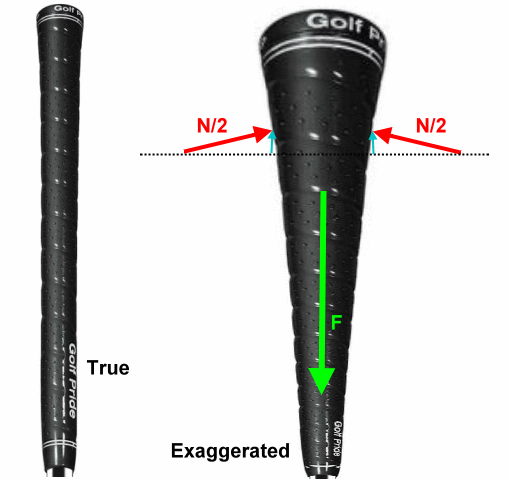The picture shows two views (one natural and one distorted) of a Golf Pride Tour Wrap grip, probably the most popular grip of all time. The idea of tapering the handle almost certainly originated from a desire to do better than just friction in letting the hands apply a centripetal force.

Look at the exaggerated view, where we have increased the butt diameter to artificially increase the amount of taper. I did this so the force vectors can be seen. (This is really a 2D approximation, so we have split N into two forces, one on each side of the grip.) Note that the normal force N, which by definition is normal to the surface, is slanted in such a way as to oppose F, the centrifugal reaction force pulling the club out of the golfer's hands. With a taper, N has a component parallel to the axis of the shaft -- the blue vector. And the blue force is opposing the centrifugal reaction force, and helping the friction force. That friction force is due to N and a coefficient of friction between the hands and the handle.

If the angle of taper is a, then the blue helping forces h amount to:

h  =  2 N sin(a/2)  ≅  N sin(a)

When we look at the true likeness of the Tour Wrap grip, we find that the taper angle is only 4° at its steepest, so:
• The small-angle approximation above is certainly valid for the Tour Wrap, and
• The total help given to the normal force N by the taper is about 7%.
While 7% is not a huge gain, it is not trivial either. It is probably worth tapering a grip to earn a 7% discount on the grip force we need to apply. So, for a tapered grip, N can be reduced to N[1-sin(a)].

Research and even simple observation tell us that most of the centripetal force on the grip near impact is exerted by the lead hand. So we should focus on the angle of taper under the lead hand. And in fact, that is where the grip taper is almost always the strongest.

Another argument I once saw for a tapered grip is that it provides a fixed diameter the hands can impose to prevent any slippage at all. I don't buy this, but I'm not an anatomist. Perhaps the hands can maintain a diameter like that, but I have only heard of hands being able to impose a diameter by exerting a force -- like N.

### Grip force: the bottom line

 Club Driver graphite shaft Driver steel shaft 5-iron steel shaft Club length 45.5 44.5 38 R 42 41 34.5 Head mass M 200 200 256 Shaft mass m 50 115 120 Head speed V ↓ Total grip force (lbs) ↓ 0 0 0 0 10 1 1 2 20 4 4 6 30 8 10 14 40 15 17 25 50 23 27 39 60 33 39 57 70 45 53 77 80 59 69 100 90 74 87 127 100 92 108 157 110 111 130 190 120 132 155 226 130 155 182 265 140 180 211 308 150 207 242 353
The total normal grip force, summed (or integrated) over the area of glove-to-grip contact, is in this table. If it looks remarkably like the table of centripetal force earlier in the article, that is no coincidence. It is a table for the same clubs at the same clubhead speeds; the only difference is that the entry is the total grip force instead of the centripetal force.

That transformation, from centripetal force to the total force the hands exert on the grip, boils down to a simple one:
• Divide the centripetal force by the static coefficient of friction, and
• Multiply by [1-sin(a)], where a is the angle of taper under the lead hand.
This table is computed using a coefficient of friction of 0.7 and a taper angle of 4°, both of which should be fairly typical. With those numbers, the total force is a third larger than the centripetal force.

There is a graph of this table in the Appendix.

## Measured human grip strength

Up to this point, we have been modeling the force needed to swing the golf club.
Now let's consider the force available. How much grip strength can the golfer actually apply, and is it enough?
Finally, in the next section, we will compare our results to experimental studies of the force actually exerted in a golf swing.

### What is grip pressure and why do we care?

I don't know whether you noticed, but every time I started to get specific -- for instance, to compute anything -- I talked about force instead of pressure. In fact, they are different, and the answers to any of the quantitative questions have answers of force, not pressure. Let's look at the difference so we can understand why all the answers are forces.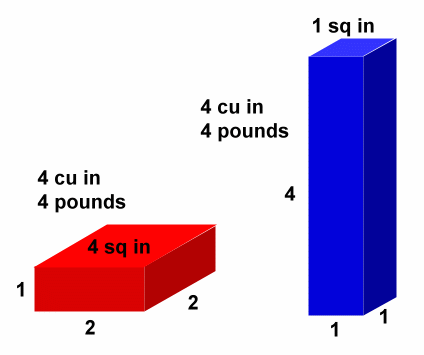In physics and engineering, pressure is the force per unit area. If you double the area but keep the pressure constant, then you double the force. That is very important in hydraulic and pneumatic mechanisms -- it is the basis of how they work -- but it is also an important distinction here. For instance, we measure force in pounds, but pressure is PSI, pounds per square inch. (In the metric system, force is Newtons, and pressure is Newtons per square meter, also called Pascals.)

Here is a picture that may make things clearer. (I can hope so, anyway.) It shows two rectangular prisms with their dimensions and other physical properties. They are the same weight and volume. Let's see what pressure each one exerts on the surface it stis on.
• The red shape sits on a 2x2 inch "footprint"; that's 4 square inches. Pressure is force per unit area. So its 4 pounds of weight apply a pressure of 4 pounds divided by 4 square inches, or 1psi (pounds per square inch) of pressure.
• The blue shape sits on a 1x1 inch "footprint"; that's 1 square inch. So its 4 pounds of weight apply a pressure of 4 pounds divided by 1 square inch, or 4psi of pressure.
.
So now we know a bit about what grip pressure is. We already know the force the hands have to exert on the grip. Can't we convert that to grip pressure by dividing by the area where the hands meet the handle? There are several things standing in the way of coming up with a useful number that way.
• There are big hands and little hands, and a whole spectrum of sizes in between. We would have to quantify the size of the hand before we had an area we could divide by.
• There are interlock grips, overlap grips, ten-finger grips, and other more obscure grip styles. This provides another variability in the area of the hands on the handle.
• So far, we are assuming constant pressure wherever the hands meet the handle. That is an incorrect assumption. Here is a pair of hands after executing a golf swing, taped with Pressurex Film,. (It comes from a study we will discuss below.)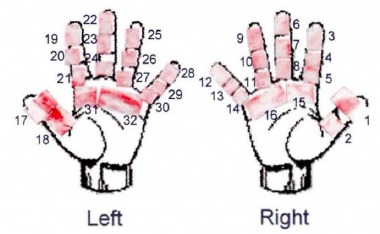The thing to note here is the extreme variation in the amount of pressure on various parts of each hand. The left thumb and the pad just under the third, fourth, and fifth fingers show a lot of pressure, while the tip of the right forefinger shows almost none. Also, the right hand (the trail hand for this test) sees far less pressure in the golf swing than the left. In order to compute the total force exerted, we would have to integrate (sum up) the force at each spot times the size of the spot.
So there is no single number representing the pressure. If you insist on a single number, you might choose the average pressure, or the maximum, or the average on each hand, or... But they would all give different numbers, and then you'd have to explain which number you chose and why. No, there is no single number that usefully represents the pressure. So let's stick with total hand force.

Why should we care about grip pressure (or force)? Just grab the club, tightly enough and then some, and go after the ball with it. The reason is that a tight grip and the tense forearms required to produce it has a bad effect on the shot. at the very least, it reduces clubhead speed. It is also easily argued that it has the same effect as trying to manipulate the club with the hands; it hurts consistency and accuracy.

Why should it reduce clubhead speed? That doesn't make sense. A tighter grip should let you use more force to swing the club. In fact, our graphs show the force increasing with clubhead speed.

Let's start with the graphs. Don't confuse cause and effect. The increase of maximum force with clubhead speed is not because the additional force produces the speed. Rather, it is because additional clubhead speed requires additional centripetal force to prevent the club from flying out of the hands. The force did not produce the speed, but is needed because the speed is there.

Now let's look at why a tight grip might reduce clubhead speed. Instructors know it does; here's one of many videos around supporting the notion. The reason boils down to a debate in the biomechanics world about whether hand torque is positive or negative at impact. The large majority of biomechanists have concluded that the hand torque coming into impact is negative; that is, it is slowing the club down. The club is moving so fast that the hands can't keep up with it. If that is true, then any effort the hands try to exert late in the downswing is counterproductive; since the hands can't keep up, they will slow the clubhead as it approaches impact. The best we can do is relax our hands, wrists, and forearms as much as possible. A corollary to this advice is to use as little grip force as we can get away with, because applying grip force with the hands will tense up the grip, make it less like the ideal frictionless hinge it should try to be.

Which brings me to the real value of hand strength. Between writing the previous paragraph and this one, I took a lunch break and caught up with a biomechanics forum where I hang out. What was freshly posted there came from a past long drive champion (Monte Scheinblum, 1992 US champion). It was so spot-on for this point, let me splice together a couple of sentences from the thread so it stands on its own:
The player has to be capable of withstanding a great deal of outward force from the clubhead’s momentum near impact, which is why I believe my ability to create high speeds is greatly attributed to my 100th percentile hand strength.
I responded:
Yes, but cause and effect get a little muddied here. The hand strength, rather than helping create the speed, allows you to hang onto the club. BUT... Yeah, it also helps create the speed, because your hands, wrists, and forearms can be more relaxed when exerting the force necessary to hang on.
Monte's reply was that he agreed 100%.

The significance is that Monte can use a much smaller fraction of his strength capability to prevent the club from flying off. With all that hand strength left in reserve, he can relax his forearms, wrists, and hands at a higher speed than you or I could while exerting the force necessary to hang onto the club.

Having established that hand strength is a producer of clubhead speed -- but in a surprising and unexpected way -- let's look at what hand strength is for typical golfers and then how that relates to the grip force that needs to be applied.

### Hand force of real golfersChris Daigle, who started me off on this, pointed me to a video that discusses the hand force in a golf grip and exercises to improve it. Hand force is measured by an instrument called a dynamometer. You squeeze it and it measures the force you apply, much like a scale measures weight. In fact, exactly like a scale measures weight.

You grip the dynamometer between your four fingers and the base of the thumb. The fingers press down with some amount of force. (In the picture, it is 100 pounds for the combined force of all four fingers.) The base of the thumb presses upward with the same force. We know it is the same force because F=ma and the dynamometer isn't going anywhere. It isn't accelerating, so the net force must be zero.

With this combination of forces, the dynamometer will indicate 100 pounds of grip force.

(Actually, it isn't exactly the same force. That hand also has to cover the weight of the dynamometer itself, or it will fall to the floor. But it is orders of magnitude less than the grip force.)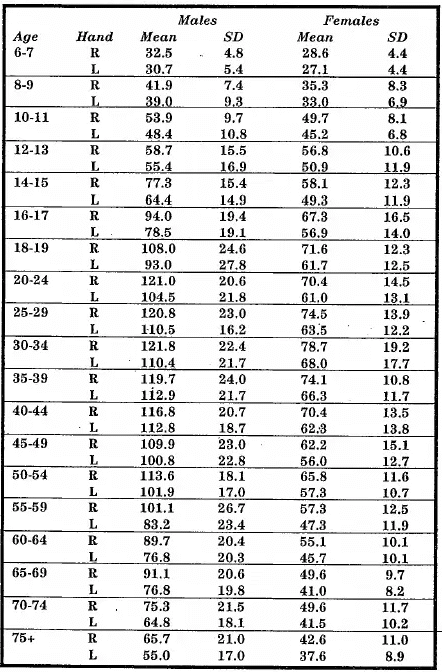Here is a table of the grip strength distribution in some population, I'm not sure what. The source is the user manual for a JAMAR brand dynamometer.

Here are a few things that jump off the chart at me:
1. It is not terribly surprising that the right hand is stronger than the left. You would expect the dominant hand to be stronger, and right-handers constitute a substantial majority of the population.
2. This RH-LH relationship is not what you would want to see. The picture of grip pressure on the gloves say that most of the gripping force is done by the lead, or non-dominant hand. We will see how much more later, but it is really a dramatic difference. Near impact, almost the whole grip force is applied by the left hand.
3. For men, the age for highest lead-hand grip strength is 35-39 years old. At that age, the average male has a 113-pound grip in the lead hand. At age 30-34, the average female can generate 68 pounds of left hand grip strength.
4. In the quote above, long drive champion Monte Scheinblum claims to have 100th-percentile grip strength. Let's assume this translates to the 3-sigma point on the bell curve, which would be the top 0.1% of the population. At age 35-39, the average lead-hand strength is 112.9 pounds and the standard deviation (one sigma) is 21.7 pounds. So Monte would have a lead-hand strength of 178 pounds (112.9+3*21.7).

Are these numbers -- the measured grip strength -- consistent with the required grip force for the clubhead speeds we know golfers attain? Let's look at three data points, using a graphite-shaft driver. We will use a split between lead hand and trail hand at impact of 95-5; I got that proportioning from measurements which we will discuss in the next section. That means full strength for the left hand and 5/95=0.053 strength for the right.

 Golfer Grip strength L + .053 R Corresponding clubhead speed Comments 37 year old male (avg strength) 119 lb 114 mph This is tour clubhead speed. So the average golfer has enough strength 33 year old female (avg strength) 72 lb 89 mph The average female golfer probably isn't close to this. But LPGA tour does better. Monte Scheinblum assumed 37yo (3-sigma strength) 188 lb 143 mph In 1992, when Monte was champion, 143mph would have been enough. Not today.

At first blush, this would appear to be a successful sanity test. But notice that there is not a lot of margin, a lot of "headroom". The golfer needs to use 80-90% of their grip strength to keep the club from flying off. Think about how much effort you have to put in to get a dynamometer to 80-90% of your maximum reading. That does not square with relaxes forearms and wrists. You may be able to hang onto the club, but it is unlikely that you can get the club to the speed you are otherwise capable of.

I conclude that the grip pressure is just too high for the grip strengths in the table. How can we resolve this dilemma?

Let's remember that the grip strengths in the table are dynamometer readings. Let's also remember how dynamometer readings relate to the forces the hands are exerting. Remembering this, let's look at an over-simplified view of the forces involved.On the left, we see the grip pressure represented by six forces of 20 pounds each, all normal to the surface of the grip. Oversimplified for tutorial purposes, not because this is a useful model of the grip. What we have is a total normal force of 120 pounds available to create friction that will exert a centripetal force.

On the right we have the same six forces, but we are going to analyze them differently. We are looking for the net force in a vertical direction represented by the yellow dash-dot line. So we have to resolve each force into its components and find the component parallel to the yellow line. That turns out to be 40 pounds downward (red vectors) balancing out 40 pounds upward (blue vectors).

It is the latter model that is closer to how a dynamometer works. The fingers press down on one side, the thumb pad presses up on the other, and the instrument measures the pressure in between. The number that pops up is the red numbers in the diagram on the right. (That is numerically the same as the blue numbers; the upward and downward forces are equal, or the dynamometer would accelerate off somewhere.)

So the dynamometer is reporting only a third of the total normal force the hand can exert. Of course, the diagrams oversimplify reality; the factor is probably less than three. But it is certainly more than two, because the hand must exert both the upward and the downward forces on the grip. So the dynamometer understates the total normal force the hand can exert by a factor of between two and three, probably closer to two.

That makes more sense. It means that the golfer is exerting less than half his or her grip strength to keep the club from flying off, which makes for less speed-robbing tension. Of course, the golfer might still use too tight and too tense a grip -- but at least we know now it is not necessary to do so, and not advantageous either.

## Measurement of grip pressure

Let's move on to actual observations of grip force during the golf swing. In 2007, Erin Schmidt published a PhD thesis on the subject (Schmidt, Erin R. (2007): Measurement of grip force and evaluation of its role in a golf shot. Loughborough University. Thesis. https://hdl.handle.net/2134/13125). Most of the measurements were done by electronic pressure sensors, specifically arrays of sensors on the gloves and on the grip of the club.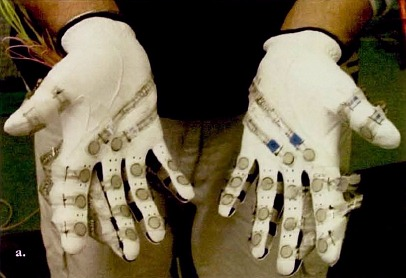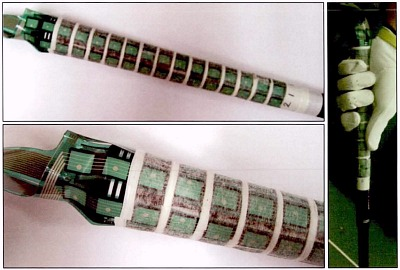For us though, the point is not the details of the instrumentation, but what we can learn about grip pressure during the golf swing. Here is Schmidt's Figure 8-2, which I have annotated to identify things described in the caption and text of the source document. The graph is the total grip force (the sum of all the glove sensors) vs time for the swing of a professional golfer. Not just any professional, but a Ryder Cup team member, so someone who is really good.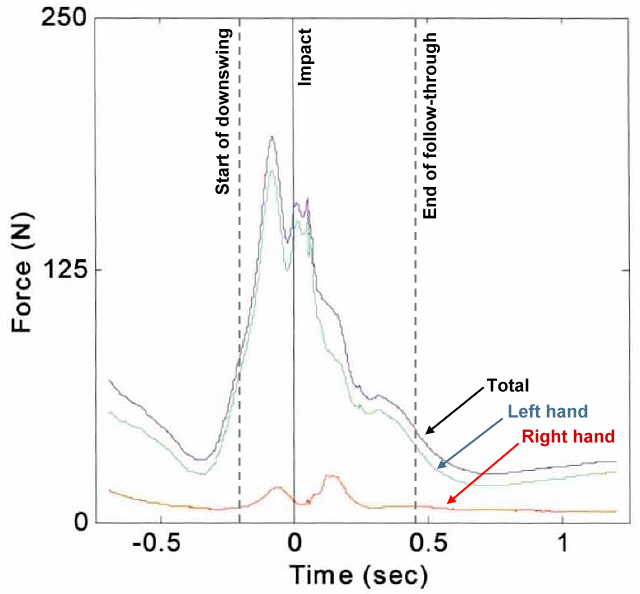I looked at this and noticed several things, some which rang true and others that made me say, "Hmmm!"
1. The force provided by the right hand, especially in the downswing where the forces are high, is a very small fraction of the total force, just a shade over 5% near impact.
2. The total force near impact is only 160N, or 36 pounds. Hmmm! In order to exert the centripetal force to hold onto the club at a 115mph (typical pro tour) driver speed, we would need 120 pounds, more than three times what Schmidt reports.
3. The maximum force occurs about 80msec before impact, and there is a dip in total force just before impact -- exactly where we would have expected the maximum force exerted. Hmmm!
Let's discuss all three.

### 1. Very little right hand force

We should already know this, even without seeing Schmidt's graph. If you watch the tour pros play on TV, you will have noticed some big hitters that actually let go with their right hand at or just after impact. For instance, Vijay Singh, Fred Couples, and Phil Mickelson. (I don't know if any golfers from the most recent generation do, but these guys did even at their prime.) That is zero percent right hand at impact, even more extreme than the 5% we used in our calculations earlier. There is even a video by Clay Ballard explaining how and why this works.

The fact that Ballard has bothered to make a video on the subject suggests this is not known and practiced by everybody. Good golfers do it, but many beginners and duffers do not. Here is a set of force traces like the one above, but for sixteen of the amateur golfers in the study. They are presented in order of increasing handicap; the handicap index is in parentheses on the graph. While there is not a perfectly uniform increase of percent right hand force with handicap, you can notice a trend. And the two highest index golfers in the figure have right-hand contributions of more like 30% than 5%.

### 2. Not enough total force

At impact, 36 pounds is not nearly enough to keep the club from flying out of our hands; that would be more like 120 pounds. How is it even possible to measure such a low number?

Remember that Schmidt had two different types of measurements: sensors on the gloves and sensors on the grip. The separate-hands graph above was made from glove measurements. If we compare it with graphs from the grip sensors, we will find a similar shape but a very different scale.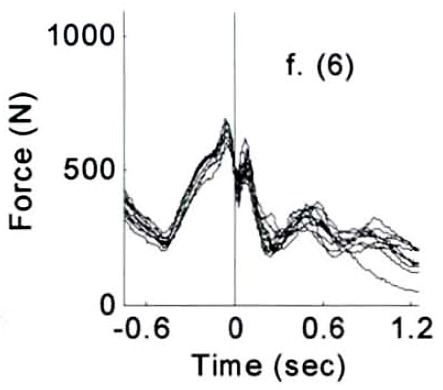Unfortunately, Schmidt did not capture the swing of the Ryder Cupper using the grip sensors, just the glove sensors. So I can't compare the two instruments with the same swing. As my "plan B"I have chosen one trace from a display of grip sensor data of multiple golfers. I chose the trace that looked to my eye to be most like the shape of the professional. It turned out to be a 6-handicap golfer. (The multiple traces on the graph reflect a different point Schmidt was making: the swing of any one golfer is a "signature" that doesn't vary much from swing to swing.)

In this trace, the force at impact is about 500N, or 113 pounds. That is much closer to the 120 pounds we need to keep the clubhead from flying off. The difference might be experimental, or it might be due to a very understandable difference in clubhead speed between the single-digit amateur and the Ryder Cup team professional. Depending on which explanation is correct, there is a factor of 3.1 or 3.3 between the glove sensors and the grip sensors. For the rest of our interpretation, let's assume a compromise value of 3.2.

We still ought to try to understand why there is a difference between the glove sensor and the grip sensor. Schmidt has noted differences in the area covered by the sensors. That is a credible explanation; if you look at the pictures of the glove and the grip above, the grip has a much larger percentage of the area covered. So the glove might be applying forces to the grip through a surface other than the sensor itself. I suspect that could completely explain the difference.

So why use an instrumented glove at all, if its reported force is a factor of more than 3 on the low side? The answer is detail. Only with the glove sensors can you assign a force to each finger, and even a LH-RH assignment may be less than 100% reliable from an instrumented grip.

We will need this full understanding as we go on to the next "Hmmm!"

### 3. Maximum force 80 milliseconds before impact

The maximum total force for the professional golfer occurred about 60% of the way through the downswing, a full 80msec before impact. That is when the torque accelerating the club is changing from the hand couple to the inertial moment of the force. The centripetal force being exerted by the hands is a lot lower than at impact, because:
1. The angular velocity is so much lower than impact. This is the major reason.
2. The radius of curvature is larger than it will be at impact. Looked at a different way, a much higher percentage of the angular motion of the club is due to the rotating left arm than is the case at impact, where most of the angular velocity is due to wrist hinging..
So I was very surprised to find a greater total force on the grip 80msec before impact than that required to counter centripetal force at impact. Where is this force coming from? It certainly isn't a "hang on" force to keep the club from flying away.

All I could think of was the hand couple, perhaps at its maximum before it gave way to inertial release. Let's check this out. Fortunately, we have some actual measurements to help us:
• Schmidt has included the forces exerted by the individual fingers. This is necessarily recorded from the glove sensors, so we will have to multiply things by 3.2 when we are done.
• To quantify the hand couple, there is another paper, by Koike, measuring the hand forces on the grip during the downswing. These forces are reported as the individual hand forces and the individual hand couples.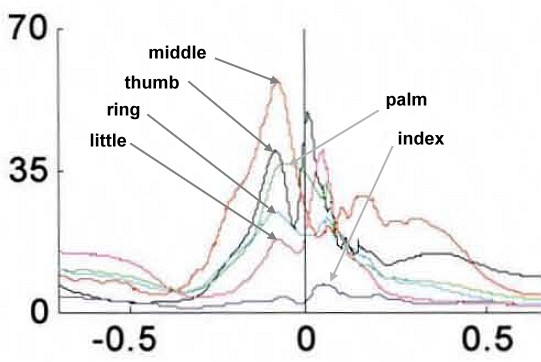Let's start with the individual finger forces of the left hand. Here is Schmidt's graph of the finger forces in Newtons, which are from the same professional swing as the hand forces shown earlier. It is very clear where the forces peak, as well as the "slot" just before impact.

The significant forces are:
• Middle finger: 60 N
• Thumb: 40 N
• Ring finger: 25 N
• Little finger: 15 N
• Palm: though significant, we will ignore it. If you look at the sensors on the glove, the palm sensors would be on the side when gripping a club (not top and bottom); the force would be almost all cross-plane and not in-plane.
• Index finger: too small to bother with, at about 5 N.
Schmidt has a similar graph for the right hand. But, as we have already seen, the right-hand forces are smaller by an order of magnitude -- so much smaller that we are not going to bother doing the detail with them that we are doing with the left. Accounting for the right hand will only add 6% or so to the totals, at least for the professional swing we are analyzing.Let's see what that looks like on a force diagram. Here are the significant forces, as identified above. The index finger exerts a very small force, and we can see that the palm is pressing perpendicularly to the other forces.

Let's see what the net force and torque the left hand is exerting on the club. The forces, of course are in red and labeled. The green line is a "ruler", with tick marks every 25mm (roughly one inch). We see that the finger forces are on the 0, 25, 50, and 100mm ticks.Let's resolve this into a net force and a couple.

The net force is easy. It is 60 N upward. (15+25+60-40)

It is harder to know where that net force is, but we will need to in order to find the equivalent couple. We separate out the upward and downward forces and find where each group would be as an equivalent single force. The downward forces are easy; there is only one of them, the 40N force. We treat the upward forces as if they were weights, and find the center of gravity of those weights. That turns out to be 64mm from the downward force. So the two solid red vectors are the equivalent upward and downward forces. They are:
• 60 N upward force.
• 40*0.064 = 2.56 Nm clockwise couple.
We mustn't forget that this set of glove sensors gives a total force roughly 3.2 times smaller than the grip sensor.) So, multiplying by 3.2, the force and torque are more likely to be:
192 N upward and 8.2 Nm in a direction to release the club.

Do we have a sanity test for this number? It turns out that we do.

A Japanese team headed by S. Koike has published several papers over the years, describing the results of studies involving an instrumented grip to determine the hand forces and hand couples during the swing. Here is an excerpt from the abstract of their 2006 paper (S. Koike, H. Iida, H, Shiraki, M. Ae, An Instrumented Grip Handle for Golf Clubs to Measure Forces and Moments Exerted by Each Hand During the Swing Motion, Engineering and Sport 6, 2006.)

An instrumented grip handle was designed to simultaneously measure the forces and moments exerted by each hand on the handle during golf swing. Eleven pairs of strain gages were attached on the surface of an aluminum bar inserted under separated grip covers... A professional golf player participated in this study and performed golf swings with several clubs.

Here is a graph of the key results in Koike's study. I have shown our left-hand cross-shaft force and torque on the graph. The red dots are the values we just computed from the Schmidt measurements, and the green dots are Koike's measurements.The good news is our numbers are within a factor of two of Koike's numbers. The bad news is:
• Our force is low by about a third and our couple low by about a half, compared with Koike's numbers.
• The numbers for the right hand are not in the same ballpark as Koike's. We wrote off the right hand forces measured by Schmidt as too small to consider. Koike has the right hand comparable to the left hand.
Let's look at each of these problems.

The first is not really that much of a problem. The factor of 3.2 was the result of eyeballing the grip sensor measurements when comparing them against the glove sensor measurements. We were further hampered by not having a grip sensor graph of the Ryder Cup professional's swing, so we were operating by guesswork. We compared the glove measurements of a top tour professional with the grip measurements of a six handicap amateur. So the actual factor might be quite different.

An even more likely explanation is our assumption that the grip sensor measurements were an accurate representation of the total grip force. But look at the instrumented grip. It is likely that there is unmeasured pressure here, just less unmeasured pressure than the glove sensor measurements. If we accounted for everything, we might get numbers close to what Koike did.

The second discrepancy, the near absence of any significant right-hand influence, is much harder to explain. Perhaps Schmidt got it right and Koike did not. Perhaps they both got it right for the population of golfers they tested. I don't know.

## Conclusion

We first found F, the centripetal force the hands need to exert on the club to keep it from flying down the fairway. Then we looked at the mechanisms by which the hands can exert an axial force on the club shaft. They consist of friction and taper. Applying these mechanisms to the formula for F, we get the following expression for G, the total force in pounds the hands exert on the grip.

 G  = 0.00129 V2  μs R (M + m/2) [1 - sin(a)]

Where:
• V is the clubhead speed, in miles per hour.
• μs is the static coefficient of friction between the hands and the handle..
• R is the distance from the mid-hands point to the center of mass of the clubhead, in inches.
• M is the mass of the clubhead, in grams.
• m is the mass of the shaft, in grams.
• a is the angle of taper of the grip where the hands are applying the force.
This is not necessarily the largest force the hands exert on the grip during the downswing, but it is the largest one where loss of the club would be the consequence of not having enough hand strength. The cross-shaft force exerted earlier in the downswing -- at the beginning of release -- may actually call for an even larger total force. Whether it does varies considerably from golfer to golfer. But, for that force, the hands push and pull instead of squeeze.

## Appendix

### Shaft with a non-uniform mass distribution

Math stuff. You won't miss much if you don't bother reading this. But if you're an engineer, you might enjoy it.

This is the derivation of the centripetal force of the shaft, where the mass distribution is arbitrary. The centripetal force turns out to depend only on where that distribution places the balance point of the shaft. We will refer to the diagram we used for the same calculation with a uniform distribution.

Let us begin by exploring the balance point. We define the distance from mid-hands to the balance point as B, and the arbitrary mass density along the shaft as D(r). B is calculated as:

 B  = 1 m R ∫ r=0 r D(r) dr

Every engineer should know this off the top of their head. You did dozens of homework problems in school based on this formula. Remember this; we'll get back to it at the end.

Now let's take our original formula for the centripetal force on the shaft, slightly reformulated for an arbitrary D(r). Specifically, we know that dm=D(r)dr.

 F  = R ∫ r=0 v2 dm r = R ∫ r=0 v2 D(r) dr r

Plugging in  v=(V/R)r  from our earlier work, we get:

 F  = R ∫ r=0 r2 VR2 D(r) dr R2 r = VR2 R2 R ∫ r=0 r D(r) dr

Now let's get clever and multiply both numerator and denominator by the shaft mass m.This should not change anything.

 F  = m VR2 R2 ( 1 m R ∫ r=0 r D(r) dr )

We should recognize the part inside parentheses as the balance point distance B. So the centripetal force becomes:

 F  = m VR2 R B R

That is, the centripetal force is a fraction of the force from a point mass at the tip of the shaft (the first factor), that fraction being B/R. If the shaft has a uniform mass distribution, then the balance point is in the middle and B/R is one half -- the result we got earlier.

### Graph of centripetal force vs clubhead speed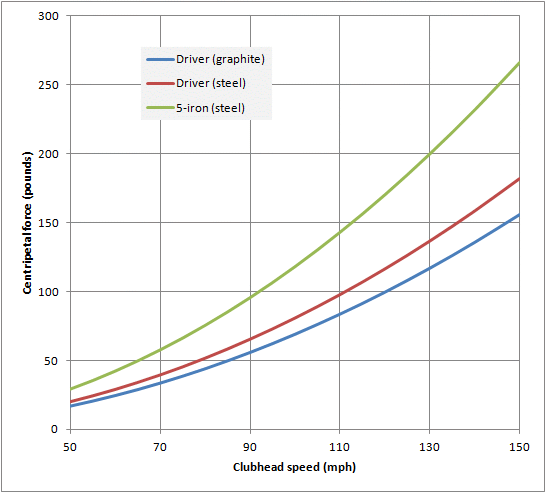### Graph of grip force vs clubhead speed